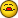# Adding numbers from other sheets?

U

#### Unforgiving

I have 5 cells on 5 sheets to add numbers together giving me a total on the
6th sheet cell, that formula is working just fine.
But I need to pull the total from 6th sheet cell into a 7th sheet giving me
the total I have on the 6th sheet cell, but when I put in my formula to pull
it into the 7th sheet it gives me one number less than what I need and I for
the life of me figure out whats going wrong.
my formula to pull the total into the 7th sheet for this is as follows:
=SUM('[Totals]Grand
Totals'!J2)+(D7)+(E7)+(F7)+(G7)+(H7)+(I7)+(J7)+(K7)+(L7)+(M7)+(N7)+(O7)+(P7)-(D12)-(E12)-(F12)-(G12)-(H12)-(I12)-(J12)-(K12)-(L12)-(M12)-(N12)-(O12)-(P12)

If more info is need just let me know. I tried to as clear as I could

J

#### Joel

I don' tknow hwat is wrong, but simplifing your formula may help

=SUM('[Totals]Grand Totals'!J2)+Sum(D77)-Sum(D1212)

B

#### Bob Phillips

or even

=SUM('[Totals]Grand Totals'!J2,D77)-SUM(D1212)

--
__________________________________
HTH

Bob

Joel said:
I don' tknow hwat is wrong, but simplifing your formula may help

=SUM('[Totals]Grand Totals'!J2)+Sum(D77)-Sum(D1212)

Unforgiving said:
I have 5 cells on 5 sheets to add numbers together giving me a total on
the
6th sheet cell, that formula is working just fine.
But I need to pull the total from 6th sheet cell into a 7th sheet giving
me
the total I have on the 6th sheet cell, but when I put in my formula to
pull
it into the 7th sheet it gives me one number less than what I need and I
for
the life of me figure out whats going wrong.
my formula to pull the total into the 7th sheet for this is as follows:
=SUM('[Totals]Grand
Totals'!J2)+(D7)+(E7)+(F7)+(G7)+(H7)+(I7)+(J7)+(K7)+(L7)+(M7)+(N7)+(O7)+(P7)-(D12)-(E12)-(F12)-(G12)-(H12)-(I12)-(J12)-(K12)-(L12)-(M12)-(N12)-(O12)-(P12)

If more info is need just let me know. I tried to as clear as I could